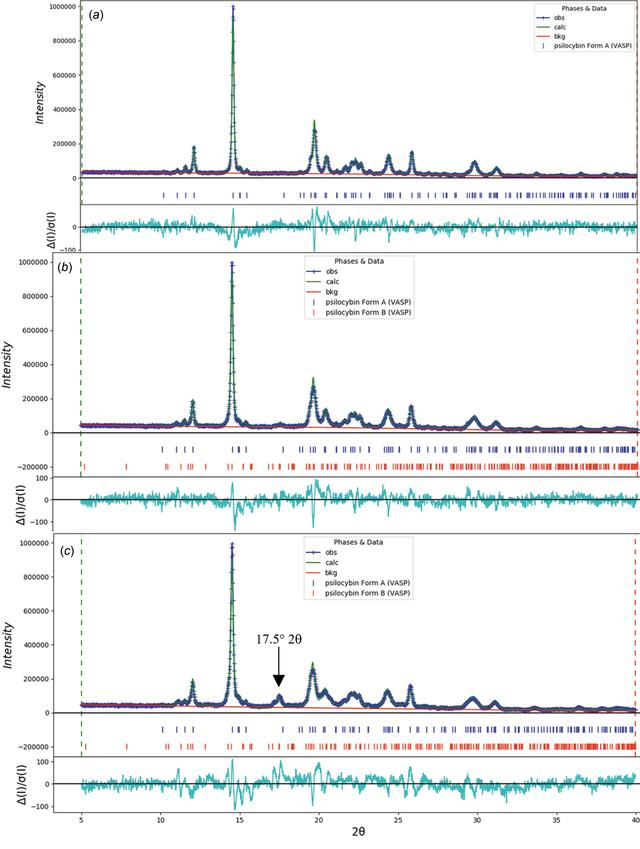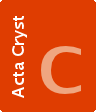disable zoom     view article Figure 24 (a) The Rietveld plot for the refinement of psilocybin Sample 22 (Hydrate A, heated 25 h at 40 °C). The blue crosses represent the observed data points and the green line is the calculated pattern. The cyan curve is the normalized error plot. (b) The Rietveld plot for the refinement of psilocybin Sample 23 (Hydrate A, heated 25 h at 45 °C). The blue crosses represent the observed data points and the green line is the calculated pattern. The cyan curve is the normalized error plot. (c) The Rietveld plot for the refinement of psilocybin Sample 24 (Hydrate A, heated 25 h at 55 °C). The blue crosses represent the observed data points and the green line is the calculated pattern. The cyan curve is the normalized error plot.STRUCTURALCHEMISTRY
ISSN: 2053-2296
Volume 78| Part 1| January 2022| Pages 36-55